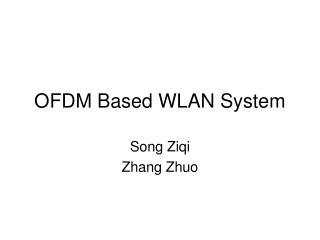DownloadDownload PresentationOFDM Based WLAN System

# OFDM Based WLAN System

Download Presentation## OFDM Based WLAN System

- - - - - - - - - - - - - - - - - - - - - - - - - - - E N D - - - - - - - - - - - - - - - - - - - - - - - - - - -
##### Presentation Transcript

1. OFDM Based WLAN System Song Ziqi Zhang Zhuo

2. Wireless Channel • Wireless channel is always unpredictable • Multipath reception is the unique characteristic of wireless channels • impairments present at the channel

3. What is Multipath • More than one transmission path between transmitter and receiver • Received signal is the sum of many versions of the transmitted signal with varying delay and attenuation

4. Effect of Multipath on Received Part • Delay Spread leads to ISI (Inter Symbol Interference) • Received signal at any time depends on a number of transmitted bits • Needs equalizer to recover data • Data Rates increase: Even WORSE!!

5. Multi-Carrier System • Single carrier system • signal representing each bit uses all of the available spectrum • Multicarrier system • available spectrum divided into many narrow bands • data is divided into parallel data streams each transmitted on a separate band

6. What is OFDM? • OFDM is a multicarrier system • uses discrete Fourier • Transform/Fast Fourier • Transform (DFT/FFT) • sin(x)/x spectra for subcarriers • Available bandwidth is divided into very many narrow bands • Data is transmitted in parallel on these bands

7. Why is OFDM? • Most broadband systems are subject to multipath transmission • Conventional solution to multipath is an equalizer in the receiver • high data rates - equalizers too complicated • With OFDM there is a simple way of dealing with multipath • relatively simple DSP algorithms

8. How does OFDM solve Multipath Problem • Data is transmitted in parallel • longer symbol period • e.g. for N parallel streams, symbol period is N times as long • Cyclic prefix • trick to avoid residual ISI

9. Transmitted in Parallel

10. Transmitted in Parallel Without Interference • Each subcarrier has a different frequency • Frequencies chosen so that an integral number of cycles in a symbol period • Signals are mathematically orthogonal

11. Cyclic Prefix

12. Disadvantages of OFDM • Strict Synchronization Requirement • Peak-to-Average Power Ratio(PAPR)

13. What shall we achieve? • Simple OFDM model including transmitter and receiver • Input random data with several modulation schemes for testing • Channel model • Demodulate the output data then compare it with the input random data

14. The OFDM Model • At the transmitter • At the receiver

15. Channel Model • Assumed to have a finite-length impulse response • Including Additive White Gaussian Noise

16. OFDM Model Design Parameters • Number of subcarriers: 64 • CP length:8 • Subcarrier frequency spacing: sample rate/64 • Modulation type per subcarrier: QPSK • Number of multipath: 5 • SNR of the channel: 30DB

17. The system

18. More • BPSK and QAM modulation • Channel Estimation • Gain and phase compensation